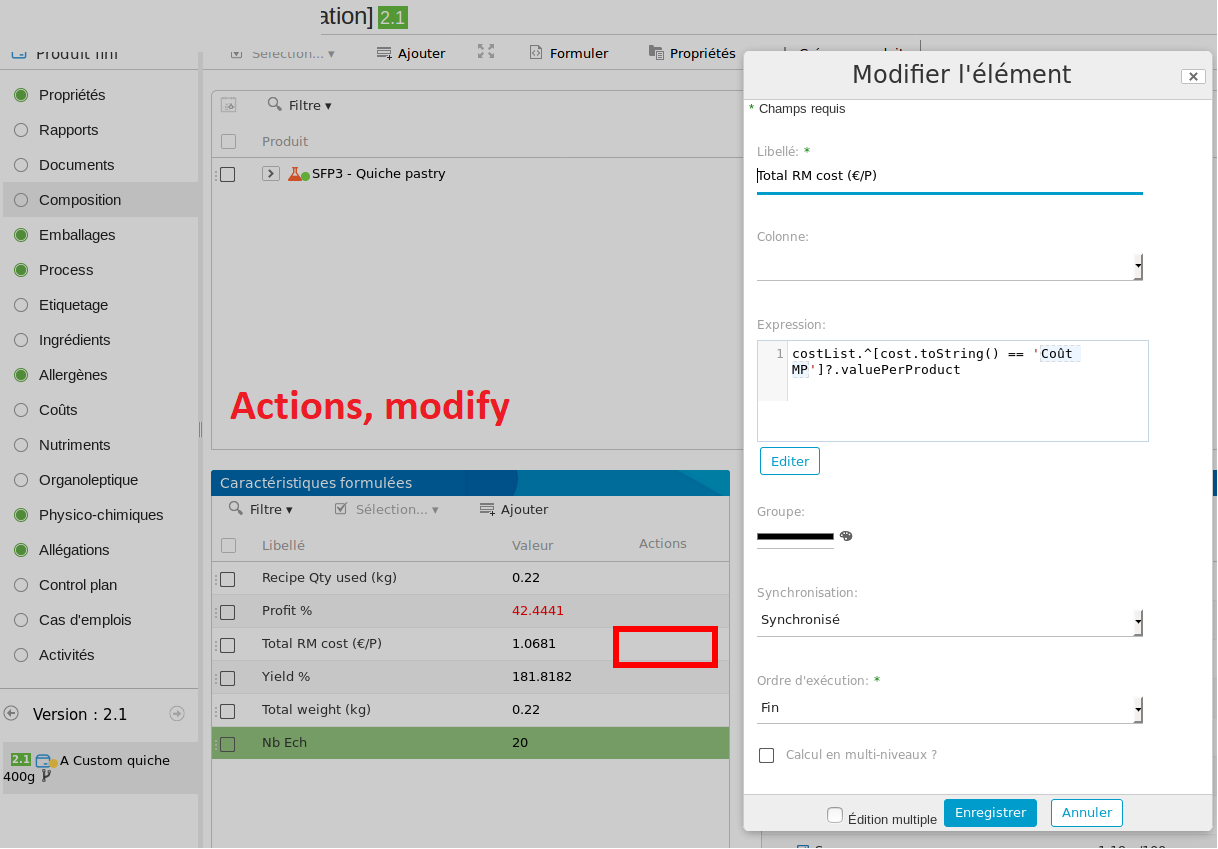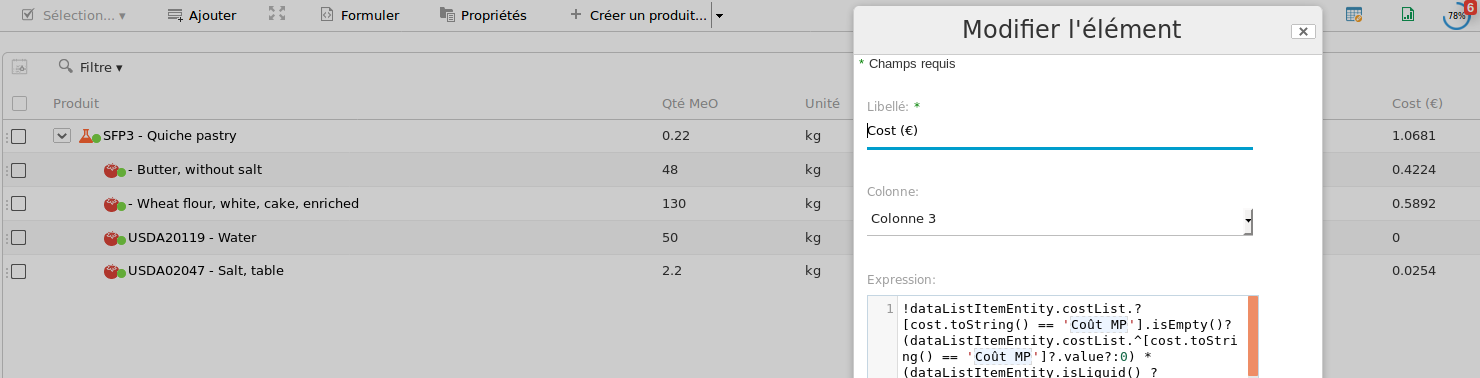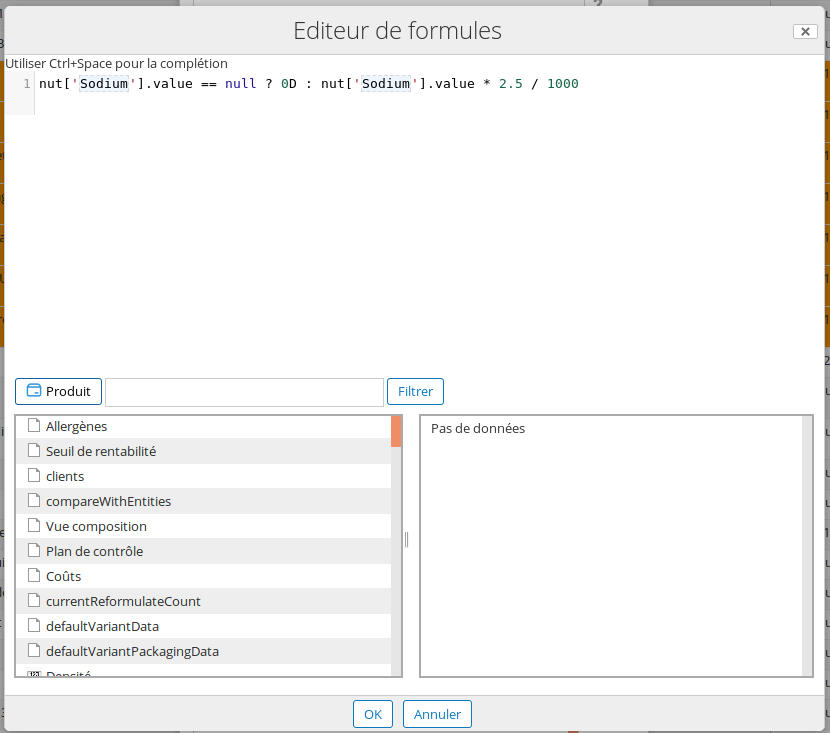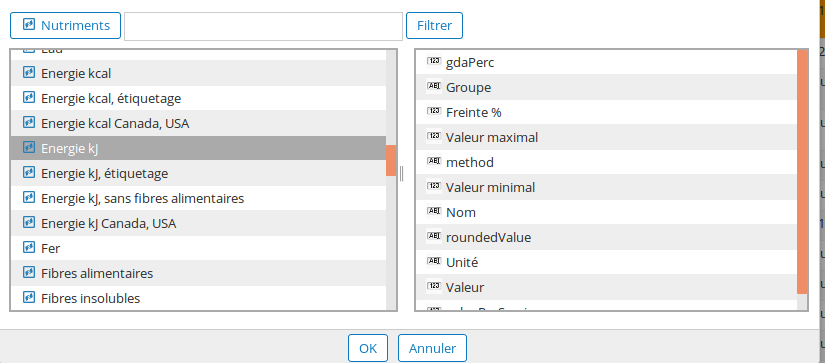Formulation often requires the use of SpEL Formulas to calculate results (for example to get the production cost of a product, or to calculate its energetic value).

This page is dedicated to the creation of these formulas in the system, and gives examples of simple and complex formulas to calculate automatically a result.

A minimum of knowledge in Java / Javascript programming is required.

# Simple formulas localization

Formulas will be found mainly in two differents locations:

• In the administration, for example for nutrients, physico-chemical characteristics,...
• On Products and product templates, in dynamic characteristicsDynamic characteristics can be done in a formulated product, or in every lines of the linked list. Every list with components (packaging, composition,process) owns its own dynamic characteristic list.

It's possible to apply a formula to all elements of a datalist by choosing a column. The results will then be displayed in a column.In this screenshot, "Costs (€)" is calculated for every components of the list.

# Generalities on a simple formula

Most of the time, SpEL formulas will display a single result; which is the last calculated expression. That means that it's possible to use intermediate variables in calculation, if the variable containing the final result is called at the end of the formula.

For example, the following formula will give the result, stocked in the `result` result

``````    var result = qty;
var yieldCoeff = (yield/100);
#result = #result / #yieldCoeff;
#result;
``````

However, most of the formulas will have only a single expression, and the use of intermediate variables won't be necessary. Then, the previous expression could be shortened:

``````    qty / (yield/100)
``````

One of the main limitation of SpEL language is the absence of conditions with `if ... else`. You need to use ternary structure which can accumulate and make the reading complex.

e.g. Salt quantity calculation (based on sodium quantity):

``````    nut['Sodium'].value == null ? 0d : nut['Sodium'].value * 2.5 / 1000
``````

(Sodium is here the nodeRef of the nutrient Sodium)

The formula first checks that the Sodium value is not null. If null, the formula displays 0. Most of the reading access to the propertu or calculation values have to include a default value, in case the value is not present. This value must have the suffix `d` (or `D`) to indicate to the sustem that this number contains comma, and not a character (example: `1d` is a number with comma, while 1 can represent the entire number 1, or the text character "1").

Multiplications work with `0d`, but divisions must have `1d`, and formulas which display text must have `""` as default value. These values must be used in condition formulas.

# Formula editor

Formula editor is presented as a text area, with numeroted lines, and two tables below.Tables under the text area enable users to quickly select fragments of formulas to insert in the text zone where the cursor is.

You can choose elements on the left, and choose the information to display from this elements at the right (value, minimumValue,...)Those variables come from ProductData class, which is used in every products, or from a list data (NutListDataItem, CostListdataItem...).Those functions come from the FormulationHelper facilitate the use of sum, multiplication, or fields value extraction.

• `entity` displays the productData type context of the formula.
• `dataListItem` displays the line of a datalist, for example a line of the composition.
• `dataListItemEntity` displays the entity (type ProductData) associated to a line of the datalist, for example the Raw Material in a composition list.
• `variantData` displays the variant linked to a line of the composition list, packaging list,...
• `@beCPG.propValue(entity, "bcpg:legalName")` displays the field Legal Name (bcpg:legalName) of the current entity.
• `@beCPG.setValue(#this, "bcpg:legalName","cm:description)` enforce the value of the field Legal Name with the value of the field Description in the current entity in this example.

Following formula can be combined:

• `@beCPG.sum(range, formula)`
• `@beCPG.avg(range, formula)`
• `@beCPG.filter(range, formula)`

To have respectively the sum, the average and the filter of a range of values.

You can also have the sum of the elements in a datalist.

``````@beCPG.sum(getCompoList(new fr.becpg.repo.variant.filters.VariantFilters(true)),dataListItem.qty)
``````

We add the filter to only consider the quantity of the composition elements with the default variant. However, we can go further and only takes in account the quantity of the componenents which are not local semi finished products.

``````@beCPG.sum(
@beCPG.filter(
getCompoList(new fr.becpg.repo.variant.filters.VariantFilters(true)),
!(dataListItemEntity instanceof T(fr.becpg.repo.product.data.LocalSemiFinishedProductData))
),
dataListItem.qty
)
``````
• `@beCPG.children(entity)` is used to get the children of `entity`, used to go down in the composition levels.
• `@beCPG.findOne(nodeRef)` is used tp get the entity designed with the `nodeRef`

Functions `idLiquid()` to `isLocalSemiFinished()` are shotcut to not use `instanceof` seen in the last formula.

# Examples of formulas

``````@beCPG.propValue(@beCPG.assocValue(entity, "bcpg:clients"), "cm:name")
``````

This fonction extracts the field Name "cm:name" of the association bcpg:clients, @beCPG.assocValue(entity, "bcpg:clients") gives its nodeRef. Useful in the case the association has a single value.

``````@beCPG.assocPropValues(nodeRef,"bcpg:clients","cm:name")
``````

This function extracts the field cm:name of the association bcpg:clients in tabular form, to treat the case of multi values in bcpg:clients field.

``````    (ingList.^[ing.toString() == 'Wheat']?.qtyPerc != null ? ingList.^[ing.toString() == 'Wheat']?.qtyPerc : 0d) +
(ingList.^[ing.toString() == 'Wheat flour']?.qtyPerc != null ? ingList.^[ing.toString() == 'Blé']?.qtyPerc : 0d)
``````

This formula calculates the sum of wheat and wheat flour % (in ingredient slist) in the product. ('Wheat' is the nodeRef, not text).

``````@beCPG.sum(getCompoList(new fr.becpg.repo.variant.filters.VariantFilters()),
"dataListItemEntity instanceof T(fr.becpg.repo.product.data.LocalSemiFinishedProductData) ? 0d : @beCPG.propValue(dataListItem.nodeRef, 'bcpg:dynamicCharactColumn2')")
``````

This formula calculates the sum of the dynamic column 2, for the components which are not local semi finished products.

``````@beCPG.sum(getCompoList(), "@beCPG.findOne(dataListItemEntity).getEntityTpl() != 'Reconstituted FP' ? @beCPG.propValue(dataListItemEntity, "bcpg:reconstitutionRate") * dataListItem.qty : dataListItemEntity.physico['Dry matter']?.value")
``````

In this formula, in following the entity model of the component, will be sum:

• The property "Reconstitution Rate", multiplied by the quantity of the composition line, if the template of the component is "Reconstituted FP".
• The physicy-chemical characteristic "Dry matter" of this component if the previous is not applicable
``````new java.text.SimpleDateFormat("dd/MM/yyyy").format(new java.util.Date())
``````

Used to get the today's date in the dd/MM/yyyy format.

``````new java.text.DecimalFormat("0.#").format(nut['Protein']?.value)
``````

Used to get personalized format on number.

``````@beCPG.propValue(\$nodeRef,"cm:description").replaceAll("toreplace","new text")
``````

Used to replace the text "to replace" to "new text" in the field cm:description.

``````entity.labelClaim['Kasher']?.isClaimed
``````

Displays true if Kosher is claimed.